Views:

Description
Besides the applied input parameters for the simulation, and the Data File summary for the output data files, the log file from a MIKE 21/3 simulation also contains details about internal simulation processes at the end of the file. This can provide useful information, e.g. when trying to optimize the simulation time. Note that the selected computer environment and eventual used GPU device is listed at the top of the same log file.

The following shows different sections in the log-file:

#01 - Hydrodynamic Simulation Diagnostic
This section shows the resulting minimum, maximum and average internal time step that was used for solving the flow equations, and the transport equations, respectively - see example in Fig. 1.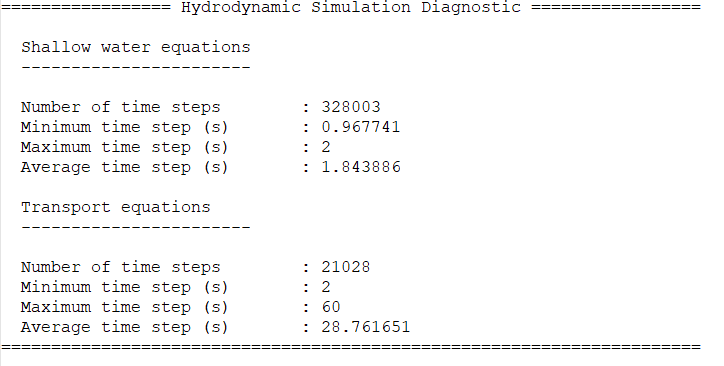Fig. 1 - Example of Hydrodynamic Simulation Diagnostic output from a MIKE 21 MT FM simulation

Note: if the resulting maximum time step is equal to the limiting maximum time step specified by the user (often similar to overall time step), then the simulation time can usually be reduced by increasing the allowed maximum time step for the simulation.

#02 - Simulation timings
The Simulation timings contains information about the time used for different groups of calculation processes in the individual modules. - see example in Fig. 2.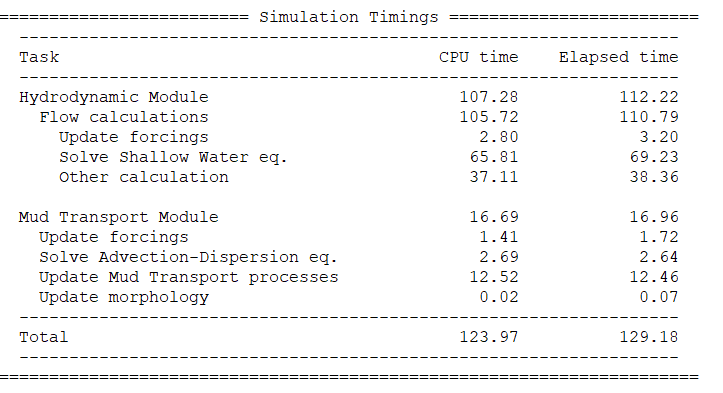Fig. 2 - Example of Simulation Timings in a MIKE 21 MT FM simulation

The timings shown are divided into ‘CPU time’ and ‘Elapsed time’.

The ‘CPU time’ is the amount of time for which the central processing units (CPU) was used for processing the instructions from the MIKE model. This is opposed to the ‘Elapsed time’, which includes e.g. waiting for input/output operations or the effect of sharing computer power and memory with other model simulations. The CPU time is the combined time for all CPUs, so when using e.g. 4 threads in parallel you might experience the Elapsed time to be smaller than the CPU time

#03 - MPI Communication Timings
For models that utilize several subdomains for the simulation, the logfile will include MPI Communication Timings. The MPI Communication timings contains information about the time used for data to be transferred between the sub-domains along the common boundaries - see example in Fig. 3.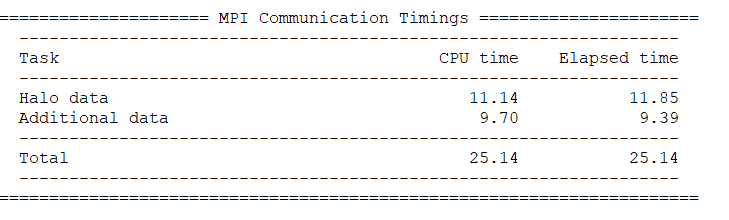Fig. 3 - Example of MPI Communication Timings output from a MIKE 21 MT FM simulation

#04 - GPU Timings
For models that utilize GPU for the hydrodynamic simulation, the logfile will include the GPU Timings.
The GPU timings contains information about the time used for different groups of calculation processes in the flow calculations. In addition, it also provides information about the time spent for Data Exchange to and from the GPU calculation - see example in Fig. 4.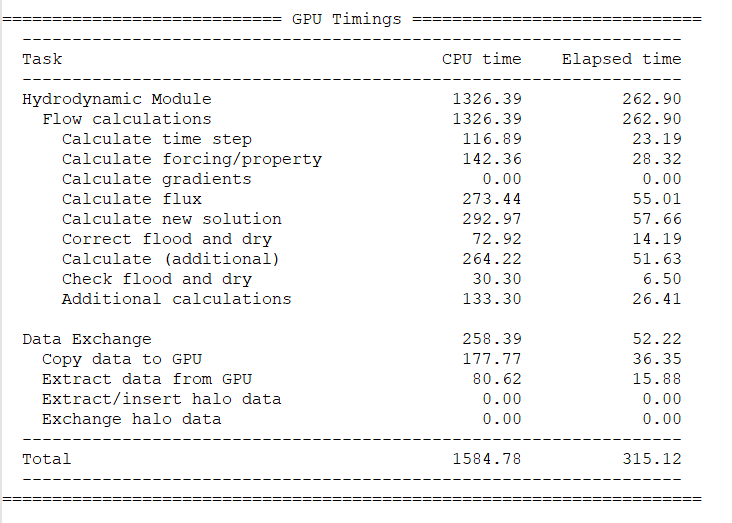Fig. 4 - Example of GPU Timings output from a MIKE 21 MT FM simulation

Note: the speed of GPU calculations depends very much on the given GPU card, and may also be affected by other things, e.g. the bandwidth between the CPU and GPU.

#05 - Overall Timings
The Overall timings sums up the time spent for the actual calculations, and the time used for pre-processing (e.g. initialization), post-processing (e.g. closing files and writing log-information) and license check during the simulation - see example in Fig. 5.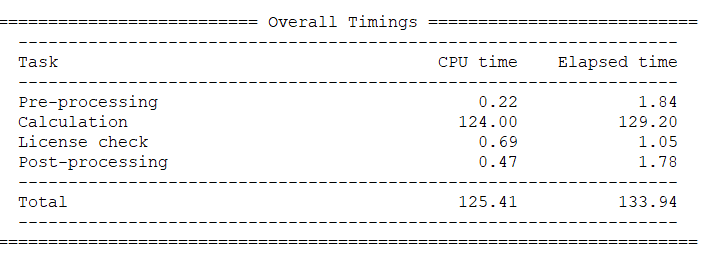Fig. 5 - Example of Overall Timings output from a MIKE 21 MT FM simulation

#06 - Memory Usage
This section displays the Peak memory usage during the simulation. In case GPU is used for the simulation, the peak memory usage is shown for CPU and GPU separately. An example is shown below.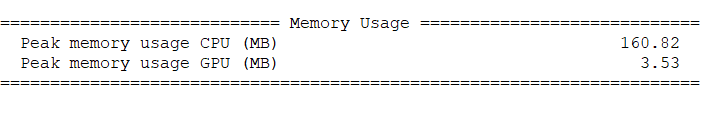Fig. 6 - Example of Memory usage a small MIKE 21 MT FM simulation

#07 - Parallelization
This section displays how the model simulation was executed, e.g. how many subdomains that were used - see example in Fig. 7.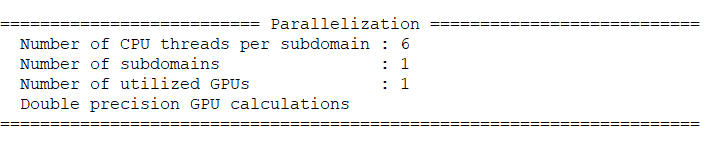Fig. 7 - Example of parallelization information for simulation